# Number – Greater & Smaller Worksheets for Grade 1

Mathematics is an important subject that promotes critical thinking and logical analysis and is the stepping stone towards independent thinking. Young children have an inherently curious mind and the right way of teaching plays a vital role in their journey of learning and education. A child’s development is guided by their experiences, understanding of concepts, forming new ideas and through interactions with the people around them. These aspects work as an integrated whole and shape the way in which young minds perceive the world around them.

One of the first concepts that are introduced to children when they begin learning mathematics is numbers. Learning numbers is a time-consuming and tedious process. However, regular practice through writing and recall is essential to ensure that children not only learn but also retain the things they are studying.

In this worksheet, we will focus on the concept of comparison of numbers and introduce the notion of greatest and smallest in numbers. The best way to familiarize children with an idea is to introduce it through verbal examples and physical demonstrations. Numbers are used in various contexts in our daily lives. Let’s look at some examples:

• There are a few books on the coffee table. Taking the books one by one, we can count the total number of books.
• There are a bunch of pencils in your pencil box. We can count the total number of pencils by taking one pencil at a time.
• Now, count the number of pencils in your friend’s pencil box. What is the number? Is it the same as the number you have? If the number of pencils in your box is 5 and the number of pencils in your friend’s box is also 5, you both have an equal number of pencils.
• Suppose the two of you do not have an equal number of pencils; your friend has 7 pencils. Therefore, your friend has more pencils or a greater number of pencils.
• Automatically, you have a lesser number of pencils than your friend.

Thus, by comparing two numbers we can easily determine which one is greater and which one is smaller. But what do we do when we have way more than just two numbers? How to compare a two-digit number with a single-digit number? In how many ways can the list of numbers be arranged?

We can easily arrange a given set of numbers from greatest to least or from least to greatest by following these rules:

• Greater the number of digits in a number, the greater the number. Therefore, a two-digit number will always be greater than a single-digit number and a three-digit number will always be greater than a two-digit number and so on. For example, 12 is a two-digit number and is greater than 7 whereas 102 is a three-digit number and is greater than both of them.
• If two numbers have the same number of digits, the digit in the left-most position must be considered. Greater the digit in the leftmost position, the greater the number. For example, 12 and 23 have the same digits but 2 is in the leftmost position of 23 and is greater than 1, which is in the leftmost position of 12. Therefore, 23 is greater than 12.
• If two numbers have the same number of digits and the leftmost digit in both the numbers are the same, then we move on to the second leftmost digit and compare them. For example, 12 and 15 have the same number of digits and they both have 1 in the leftmost position. However, 5 is in the second leftmost position in 15 and is greater than 2, which is in the second leftmost position of 12. Therefore, 15 is greater than 12.

## Greater & Smaller Worksheets for Grade 1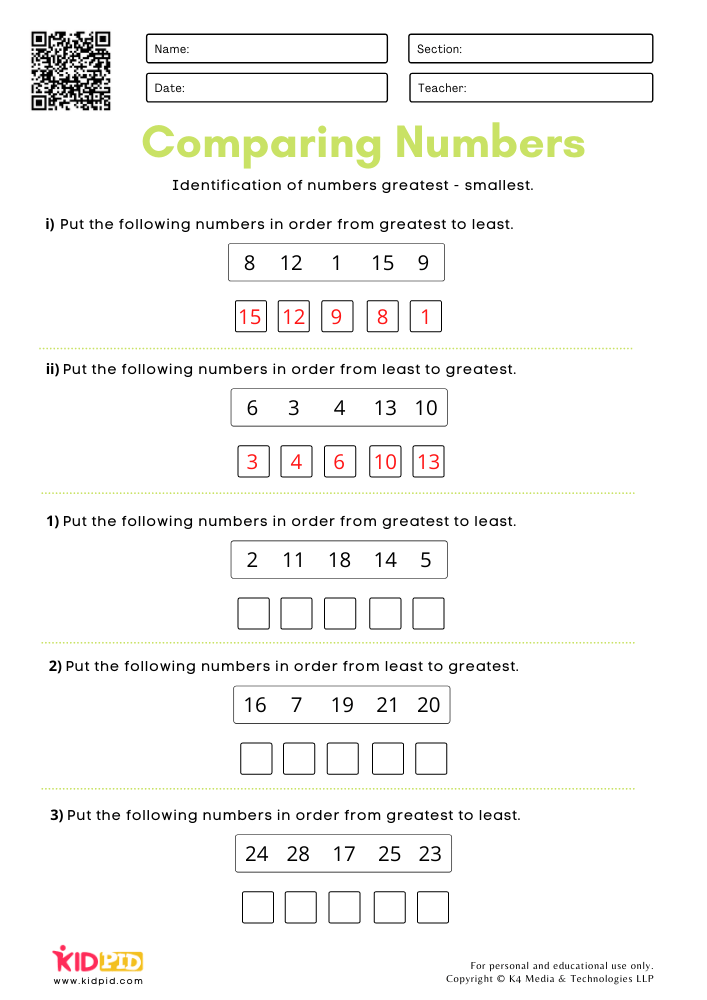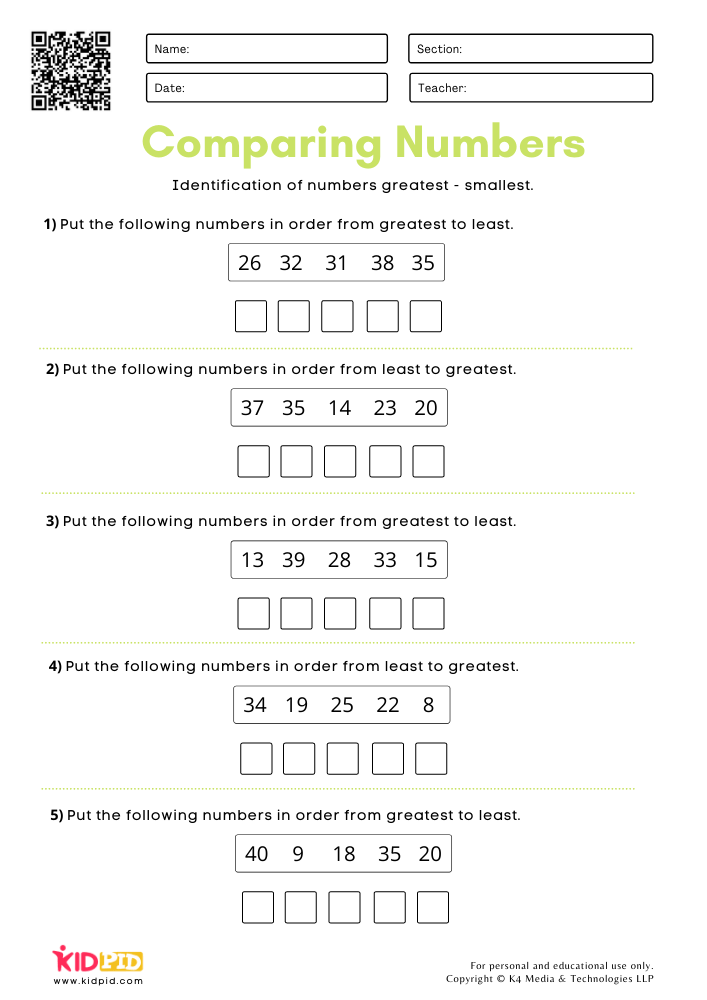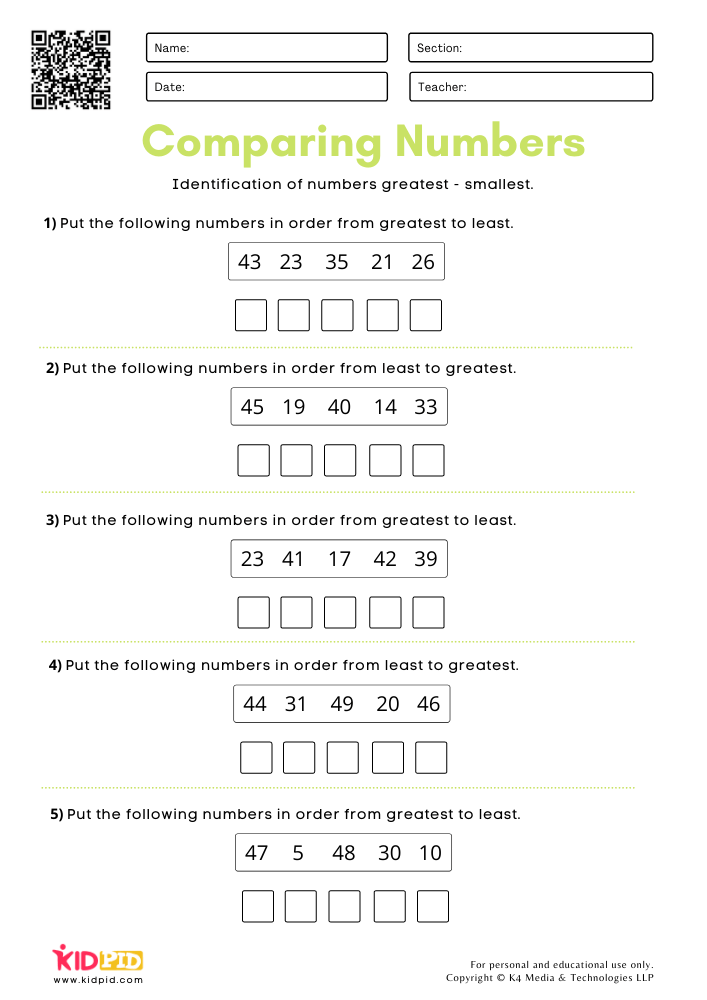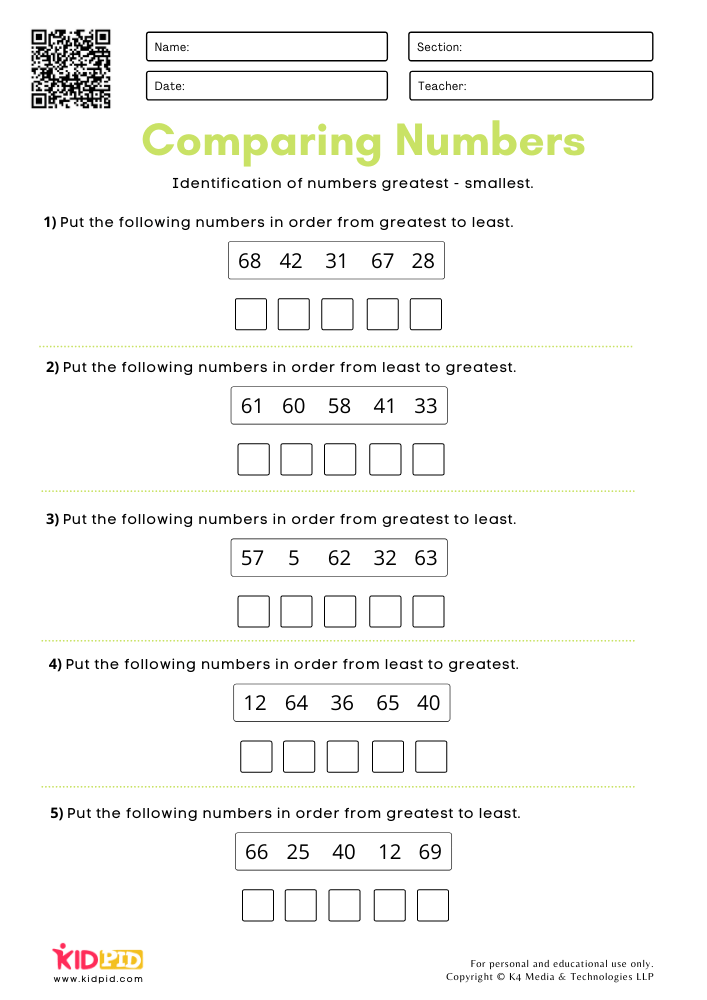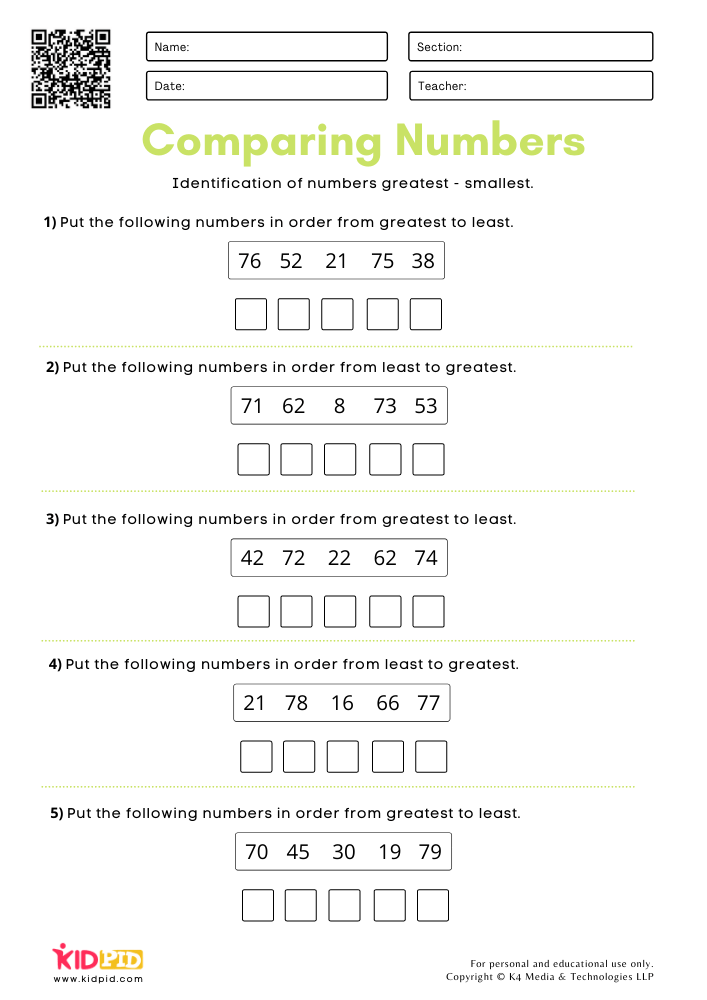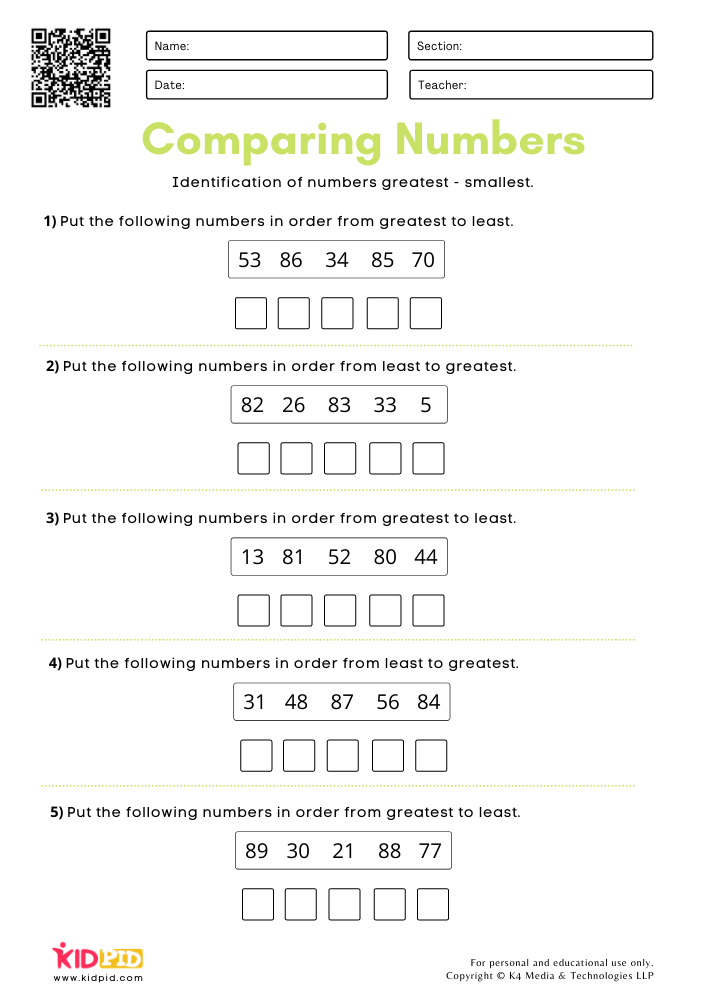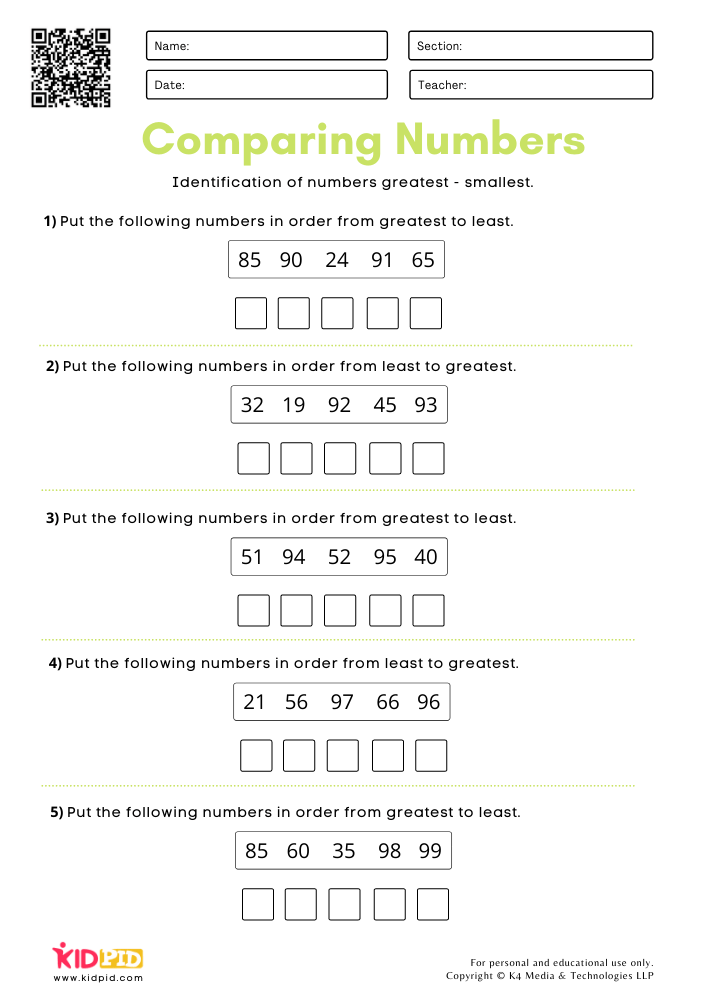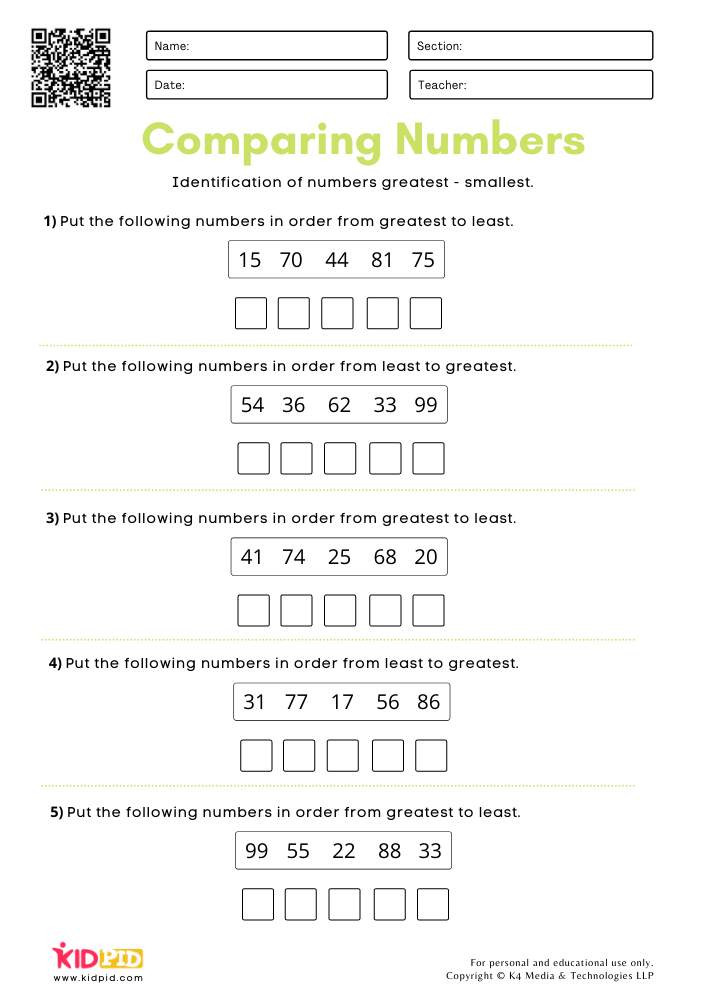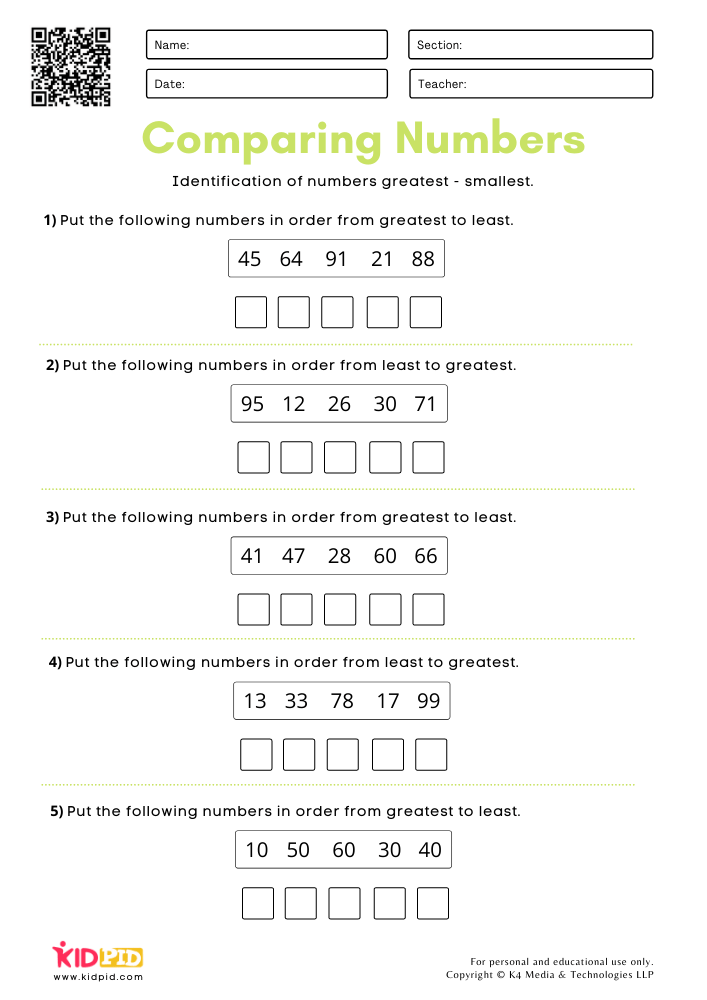These rules must be kept in mind and applied while solving the worksheet. One simply has to identify the greatest and the smallest number given in each list and arrange the numbers either from smallest to greatest or greatest to smallest. Comparing multiple numbers at the same time can be tricky and so it is important that children are focused while solving it. This exercise will not only help them brush up on numbers and counting but will also help them easily retain a newly learned concept.

So, what are you waiting for? Download your free worksheet, print it out and get started today!

## A-Z Letter Focus Worksheets for Preschool

Kindergarten is the stage where children become very curious and have a hunger for learning new things. It is crucial to stimulate and challenge them…

## How to Teach Kids About Computer in a Delightful Way

Computers are one of most seen thing in present time. They’re things we use for almost every function, to send mail, write a story, talk to…

## Fun Ways to Prepare Your Kid for Primary 1 Maths

Maths is everywhere. Maths talk is important. Math leads to critical thinking, problem-solving skill, and better reasoning abilities. It’s very important to prepare your kids…